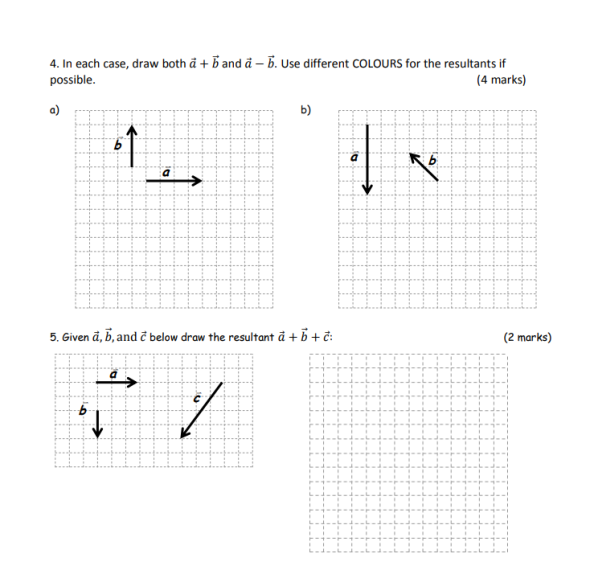# Question Solved1 Answer4. In each case, draw both a + b and å – 5. Use different COLOURS for the resultants if possible. (4 marks) b) o ] a 5. Given a, b, and below draw the resultant a + b + : (2 marks) to Ć 6 /Transcribed Image Text: 4. In each case, draw both a + b and å – 5. Use different COLOURS for the resultants if possible. (4 marks) b) o ] a 5. Given a, b, and below draw the resultant a + b + : (2 marks) to Ć 6 /
More
Transcribed Image Text: 4. In each case, draw both a + b and å – 5. Use different COLOURS for the resultants if possible. (4 marks) b) o ] a 5. Given a, b, and below draw the resultant a + b + : (2 marks) to Ć 6 /